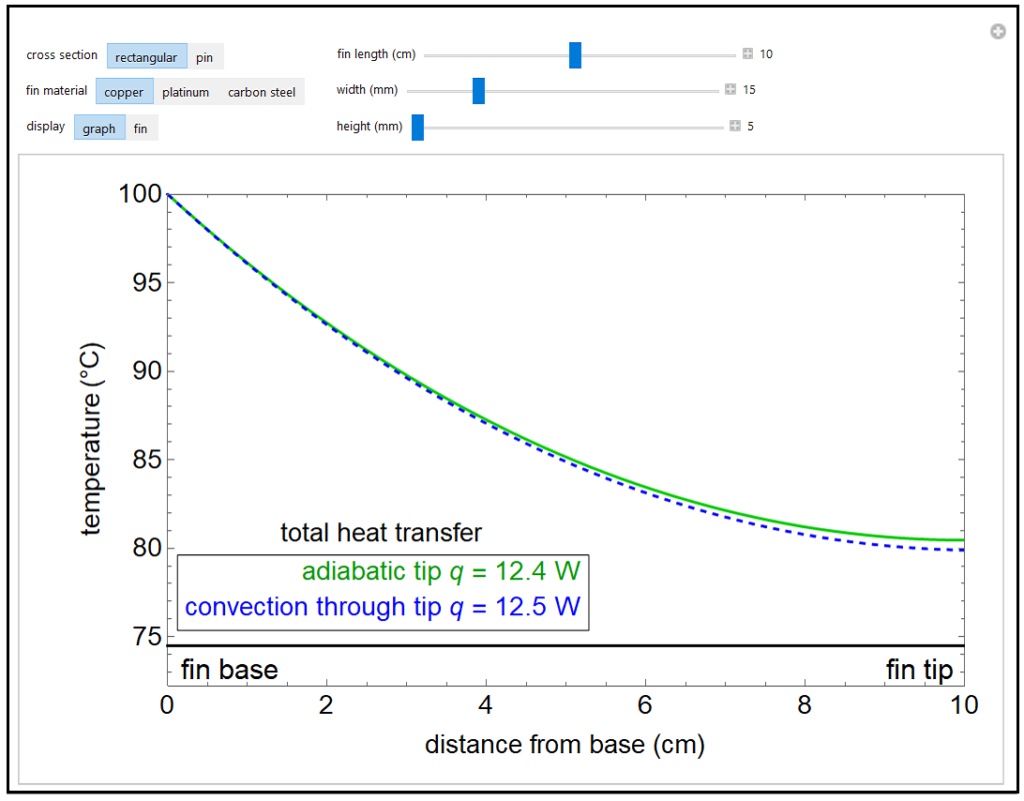LearnChemE

##### Heat Transfer and Temperature Distribution in a Fin##### Description

This simulation calculates temperature as a function of distance from the base of a heat transfer fin of uniform cross-sectional area. The fin is attached to a base, which has the same cross-sectional area and is at a constant temperature of 100°C. Heat loss from the fin is by natural convection to the surrounding air, which is at 25°C. The rate of heat transfer is calculated for an adiabatic tip (no heat transfer through the tip surface) and for a tip that has heat transfer by convection. The temperature decreases down the fin due to conduction and to heat being lost by convection. The conduction is assumed to be one-dimensional along the length of the fin. The length and diameter of the fin can be varied, and its cross section can be either rectangular or circular. Three fin materials with different thermal conductivities can be selected.

This simulation runs on desktop using the free Wolfram Player. Download the Wolfram Player here.